# 量子傅里叶变换

## 1. 简介

y_k = \frac{1}{\sqrt{N}}\sum_{j=0}^{N-1}x_j\omega_N^{jk}

y_k = \frac{1}{\sqrt{N}}\sum_{j=0}^{N-1}x_j\omega_N^{jk}

\omega_N^{jk} 定义如上所示。注意，只有状态的振幅受到这种变换的影响。

\vert j \rangle \mapsto \frac{1}{\sqrt{N}}\sum_{k=0}^{N-1}\omega_N^{jk} \vert k \rangle

U_{QFT} = \frac{1}{\sqrt{N}} \sum_{j=0}^{N-1} \sum_{k=0}^{N-1} \omega_N^{jk} \vert k \rangle \langle j \vert

## 2. 直觉

\text{QFT}|x\rangle = |\widetilde{x}\rangle

(我们经常用波浪线(~)来表示傅里叶基中的状态)

### 2.1傅里叶基计数: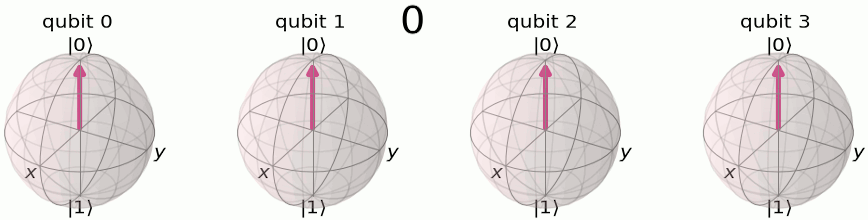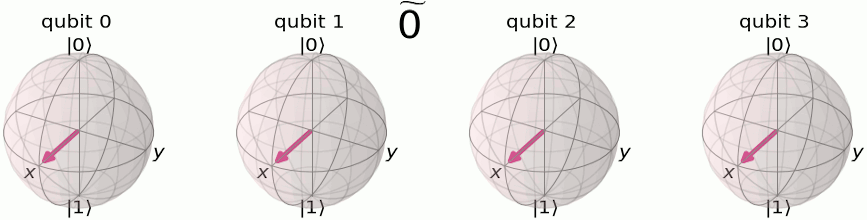## 3. 例1:1量子比特QFT

y_0 = \frac{1}{\sqrt{2}}\left( \alpha \exp\left(2\pi i\frac{0\times0}{2}\right) + \beta \exp\left(2\pi i\frac{1\times0}{2}\right) \right) = \frac{1}{\sqrt{2}}\left(\alpha + \beta\right)

y_1 = \frac{1}{\sqrt{2}}\left( \alpha \exp\left(2\pi i\frac{0\times1}{2}\right) + \beta \exp\left(2\pi i\frac{1\times1}{2}\right) \right) = \frac{1}{\sqrt{2}}\left(\alpha - \beta\right)

U_{QFT}\vert\psi\rangle = \frac{1}{\sqrt{2}}(\alpha + \beta) \vert 0 \rangle + \frac{1}{\sqrt{2}}(\alpha - \beta) \vert 1 \rangle

H = \frac{1}{\sqrt{2}}\begin{bmatrix} 1 & 1 \\ 1 & -1 \end{bmatrix}

\frac{1}{\sqrt{2}}(\alpha + \beta) \vert 0 \rangle + \frac{1}{\sqrt{2}}(\alpha - \beta) \vert 1 \rangle \equiv \tilde{\alpha}\vert 0 \rangle + \tilde{\beta}\vert 1 \rangle

## 4. 量子傅里叶变换

N更大时量子傅里叶变换是什么样的?让我们推导 N=2^ N ，$QFT_N$ 作用于状态 \vert x \rangle = \vert x_1\ldots x_n \rangle 的变换，其中 x_1 是最高位。这个数学是为那些觉得它有用的人准备的，如果你纠结于它，那么不要担心;只要你理解了第2部分中的直觉，你就可以直接进入下一部分。

\begin{aligned} QFT_N\vert x \rangle & = \frac{1}{\sqrt{N}} \sum_{y=0}^{N-1}\omega_N^{xy} \vert y \rangle \\ & = \frac{1}{\sqrt{N}} \sum_{y=0}^{N-1} e^{2 \pi i xy / 2^n} \vert y \rangle ~\text{因为}\: \omega_N^{xy} = e^{2\pi i \frac{xy}{N}} \:\text{和}\: N = 2^n \\ & = \frac{1}{\sqrt{N}} \sum_{y=0}^{N-1} e^{2 \pi i \left(\sum_{k=1}^n y_k/2^k\right) x} \vert y_1 \ldots y_n \rangle \:\text{ * 用分数二进制表示法重写}\: y = y_1\ldots y_n, y/2^n = \sum_{k=1}^n y_k/2^k \\ & = \frac{1}{\sqrt{N}} \sum_{y=0}^{N-1} \prod_{k=1}^n e^{2 \pi i x y_k/2^k } \vert y_1 \ldots y_n \rangle \:\text{将和的指数展开为指数的乘积之后} \\ & = \frac{1}{\sqrt{N}} \bigotimes_{k=1}^n \left(\vert0\rangle + e^{2 \pi i x /2^k } \vert1\rangle \right) \:\text{重新排列和积，并展开} \sum_{y=0}^{N-1} = \sum_{y_1=0}^{1}\sum_{y_2=0}^{1}\ldots\sum_{y_n=0}^{1} \\ & = \frac{1}{\sqrt{N}} \left(\vert0\rangle + e^{\frac{2\pi i}{2}x} \vert1\rangle\right) \otimes \left(\vert0\rangle + e^{\frac{2\pi i}{2^2}x} \vert1\rangle\right) \otimes \ldots \otimes \left(\vert0\rangle + e^{\frac{2\pi i}{2^{n-1}}x} \vert1\rangle\right) \otimes \left(\vert0\rangle + e^{\frac{2\pi i}{2^n}x} \vert1\rangle\right) \end{aligned}## 5. 实现QFT的电路

H\vert x_k \rangle = \frac{1}{\sqrt{2}}\left(\vert0\rangle + \exp\left(\frac{2\pi i}{2}x_k\right)\vert1\rangle\right)

CROT_k = \left[\begin{matrix} I&0\\ 0&UROT_k\\ \end{matrix}\right]

UROT_k = \left[\begin{matrix} 1&0\\ 0&\exp\left(\frac{2\pi i}{2^k}\right)\\ \end{matrix}\right]

CROT_k 对两个量子比特状态 \vert x_l x_j\rangle 的作用，其中第一个量子比特是控制，第二个量子比特是目标，由

CROT_k\vert 0x_j\rangle = \vert 0x_j\rangle

CROT_k\vert 1x_j\rangle = \exp\left( \frac{2\pi i}{2^k}x_j \right)\vert 1x_j\rangle

1. 在量子比特1上的第一个哈达玛门之后，状态从输入状态转换为
H_1\vert x_1x_2\ldots x_n\rangle = \frac{1}{\sqrt{2}} \left[\vert0\rangle + \exp\left(\frac{2\pi i}{2}x_1\right)\vert1\rangle\right] \otimes \vert x_2x_3\ldots x_n\rangle
1. 在量子位2控制的量子位1上的 UROT_2 门后，状态转换为
\frac{1}{\sqrt{2}} \left[\vert0\rangle + \exp\left(\frac{2\pi i}{2^2}x_2 + \frac{2\pi i}{2}x_1\right)\vert1\rangle\right] \otimes \vert x_2x_3\ldots x_n\rangle
1. 在量子比特 n 控制的量子比特1上应用最后一个 UROT_n 门后，状态变为
\frac{1}{\sqrt{2}} \left[\vert0\rangle + \exp\left( \frac{2\pi i}{2^n}x_n + \frac{2\pi i}{2^{n-1}}x_{n-1} + \ldots + \frac{2\pi i}{2^2}x_2 + \frac{2\pi i}{2}x_1 \right) \vert1\rangle\right] \otimes \vert x_2x_3\ldots x_n\rangle

x = 2^{n-1}x_1 + 2^{n-2}x_2 + \ldots + 2^1x_{n-1} + 2^0x_n

\frac{1}{\sqrt{2}} \left[\vert0\rangle + \exp\left( \frac{2\pi i}{2^n}x \right) \vert1\rangle\right] \otimes \vert x_2x_3\ldots x_n\rangle
1. 在对量子位 2\ldots n 应用类似的门序列后，我们发现最终状态为:
\frac{1}{\sqrt{2}} \left[\vert0\rangle + \exp\left( \frac{2\pi i}{2^n}x \right) \vert1\rangle\right] \otimes \frac{1}{\sqrt{2}} \left[\vert0\rangle + \exp\left( \frac{2\pi i}{2^{n-1}}x \right) \vert1\rangle\right] \otimes \ldots \otimes \frac{1}{\sqrt{2}} \left[\vert0\rangle + \exp\left( \frac{2\pi i}{2^{2}}x \right) \vert1\rangle\right] \otimes \frac{1}{\sqrt{2}} \left[\vert0\rangle + \exp\left( \frac{2\pi i}{2^{1}}x \right) \vert1\rangle\right]

## 6. 例2:3量子比特QFT

\vert y_3y_2y_1\rangle = QFT_8\vert x_3x_2x_1\rangle 创建电路的步骤如下:

1. \vert x_1 \rangle 应用一个哈达玛门
|\psi_1\rangle = \vert x_3\rangle \otimes \vert x_2\rangle \otimes \frac{1}{\sqrt{2}} \left[ \vert0\rangle + \exp\left(\frac{2\pi i}{2}x_1\right) \vert1\rangle\right]
1. 根据 \vert x_2\rangle ，应用 UROT_2 门到 \vert x_1\rangle
|\psi_2\rangle = \vert x_3\rangle \otimes \vert x_2\rangle \otimes \frac{1}{\sqrt{2}} \left[ \vert0\rangle + \exp\left( \frac{2\pi i}{2^2}x_2 + \frac{2\pi i}{2}x_1 \right) \vert1\rangle\right]
1. 应用 UROT_3 门到 \vert x_1\rangle ，取决于 \vert x_3\rangle
|\psi_2\rangle = \vert x_3\rangle \otimes \vert x_2\rangle \otimes \frac{1}{\sqrt{2}} \left[ \vert0\rangle + \exp\left( \frac{2\pi i}{2^2}x_2 + \frac{2\pi i}{2}x_1 \right) \vert1\rangle\right]
1. \vert x_2 \rangle 应用一个哈达玛门
|\psi_4\rangle = \vert x_3\rangle \otimes \frac{1}{\sqrt{2}} \left[ \vert0\rangle + \exp\left( \frac{2\pi i}{2}x_2 \right) \vert1\rangle\right] \otimes \frac{1}{\sqrt{2}} \left[ \vert0\rangle + \exp\left( \frac{2\pi i}{2^3}x_3 + \frac{2\pi i}{2^2}x_2 + \frac{2\pi i}{2}x_1 \right) \vert1\rangle\right]
1. 根据 \vert x_3\rangle ，应用 UROT_2 门到 \vert x_2\rangle
|\psi_5\rangle = \vert x_3\rangle \otimes \frac{1}{\sqrt{2}} \left[ \vert0\rangle + \exp\left( \frac{2\pi i}{2^2}x_3 + \frac{2\pi i}{2}x_2 \right) \vert1\rangle\right] \otimes \frac{1}{\sqrt{2}} \left[ \vert0\rangle + \exp\left( \frac{2\pi i}{2^3}x_3 + \frac{2\pi i}{2^2}x_2 + \frac{2\pi i}{2}x_1 \right) \vert1\rangle\right]
1. \vert x_3 \rangle 应用一个哈达玛门
|\psi_6\rangle = \frac{1}{\sqrt{2}} \left[ \vert0\rangle + \exp\left( \frac{2\pi i}{2}x_3 \right) \vert1\rangle\right] \otimes \frac{1}{\sqrt{2}} \left[ \vert0\rangle + \exp\left( \frac{2\pi i}{2^2}x_3 + \frac{2\pi i}{2}x_2 \right) \vert1\rangle\right] \otimes \frac{1}{\sqrt{2}} \left[ \vert0\rangle + \exp\left( \frac{2\pi i}{2^3}x_3 + \frac{2\pi i}{2^2}x_2 + \frac{2\pi i}{2}x_1 \right) \vert1\rangle\right]
1. 请记住输出状态相对于所需QFT的相反顺序。因此，我们必须反转量子比特的顺序(在这种情况下，交换 y_1y_3 )。

## 8.Qiskit实现

CP(\theta) = \begin{bmatrix} 1 & 0 & 0 & 0 \\ 0 & 1 & 0 & 0 \\ 0 & 0 & 1 & 0 \\ 0 & 0 & 0 & e^{i\theta}\end{bmatrix}

\theta = 2\pi/2^k = \pi/2^{k-1}

### 8.1关于3量子比特的例子


import numpy as np

from numpy import pi

# importing Qiskit

from qiskit import QuantumCircuit, transpile, assemble, Aer, IBMQ

from qiskit.providers.ibmq import least_busy

from qiskit.tools.monitor import job_monitor

from qiskit.visualization import plot_histogram, plot_bloch_multivector




qc = QuantumCircuit(3)




qc.h(2)

qc.draw()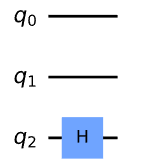qc.cp(pi/2, 1, 2) # CROT from qubit 1 to qubit 2

qc.draw()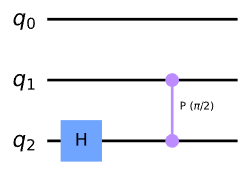qc.cp(pi/4, 0, 2) # CROT from qubit 2 to qubit 0

qc.draw()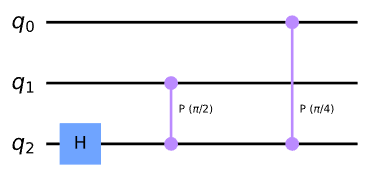qc.h(1)

qc.cp(pi/2, 0, 1) # CROT from qubit 0 to qubit 1

qc.h(0)

qc.draw()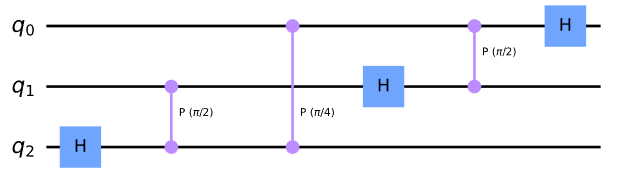qc.swap(0,2)

qc.draw()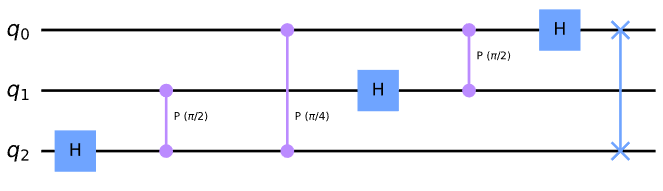### 8.2通用QFT函数


def qft_rotations(circuit, n):

if n == 0: # Exit function if circuit is empty

return circuit

n -= 1 # Indexes start from 0

circuit.h(n) # Apply the H-gate to the most significant qubit

for qubit in range(n):

# For each less significant qubit, we need to do a

# smaller-angled controlled rotation:

circuit.cp(pi/2**(n-qubit), qubit, n)




qc = QuantumCircuit(4)

qft_rotations(qc,4)

qc.draw()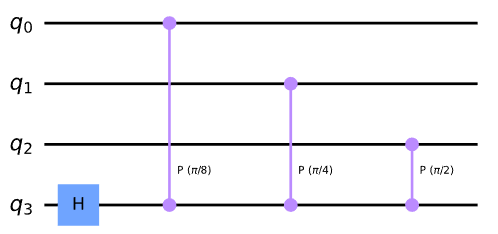from qiskit_textbook.widgets import scalable_circuit

scalable_circuit(qft_rotations)




def qft_rotations(circuit, n):

"""Performs qft on the first n qubits in circuit (without swaps)"""

if n == 0:

return circuit

n -= 1

circuit.h(n)

for qubit in range(n):

circuit.cp(pi/2**(n-qubit), qubit, n)

# At the end of our function, we call the same function again on

# the next qubits (we reduced n by one earlier in the function)

qft_rotations(circuit, n)

# Let's see how it looks:

qc = QuantumCircuit(4)

qft_rotations(qc,4)

qc.draw()




scalable_circuit(qft_rotations)




def swap_registers(circuit, n):

for qubit in range(n//2):

circuit.swap(qubit, n-qubit-1)

return circuit

def qft(circuit, n):

"""QFT on the first n qubits in circuit"""

qft_rotations(circuit, n)

swap_registers(circuit, n)

return circuit

# Let's see how it looks:

qc = QuantumCircuit(4)

qft(qc,4)

qc.draw()




scalable_circuit(qft)




bin(5)



“0 b101”

(0b提醒我们这是一个二进制数)。让我们把它编码到我们的量子比特中:


# Create the circuit

qc = QuantumCircuit(3)

# Encode the state 5

qc.x(0)

qc.x(2)

qc.draw()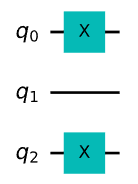sim = Aer.get_backend("aer_simulator")

qc_init = qc.copy()

qc_init.save_statevector()

statevector = sim.run(qc_init).result().get_statevector()

plot_bloch_multivector(statevector)




qft(qc,3)

qc.draw()




qc.save_statevector()

statevector = sim.run(qc).result().get_statevector()

plot_bloch_multivector(statevector)



|\widetilde{0}\rangle = |{+}{+}{+}\rangle ，量子位0旋转了 \tfrac{5}{8} 一圈，量子位1旋转了 \tfrac{10}{8} 一圈(相当于 \tfrac{1}{4} 一圈)，量子位2旋转了 \tfrac{20}{8} 一圈(相当于 \tfrac{1}{2} 一圈)。

## 8.3在真实量子设备上运行QFT


def inverse_qft(circuit, n):

"""Does the inverse QFT on the first n qubits in circuit"""

# First we create a QFT circuit of the correct size:

qft_circ = qft(QuantumCircuit(n), n)

# Then we take the inverse of this circuit

invqft_circ = qft_circ.inverse()

# And add it to the first n qubits in our existing circuit

circuit.append(invqft_circ, circuit.qubits[:n])

return circuit.decompose() # .decompose() allows us to see the individual gates




nqubits = 3

number = 5

qc = QuantumCircuit(nqubits)

for qubit in range(nqubits):

qc.h(qubit)

qc.p(number*pi/4,0)

qc.p(number*pi/2,1)

qc.p(number*pi,2)

qc.draw()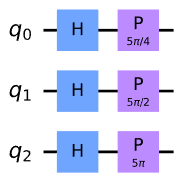qc_init = qc.copy()

qc_init.save_statevector()

sim = Aer.get_backend("aer_simulator")

statevector = sim.run(qc_init).result().get_statevector()

plot_bloch_multivector(statevector)




qc = inverse_qft(qc, nqubits)

qc.measure_all()

qc.draw()




# Load our saved IBMQ accounts and get the least busy backend device with less than or equal to nqubits

provider = IBMQ.get_provider(hub='ibm-q')

backend = least_busy(provider.backends(filters=lambda x: x.configuration().n_qubits >= nqubits

and not x.configuration().simulator

and x.status().operational==True))

print("least busy backend: ", backend)




shots = 2048

transpiled_qc = transpile(qc, backend, optimization_level=3)

job = backend.run(transpiled_qc, shots=shots)

job_monitor(job)




counts = job.result().get_counts()

plot_histogram(counts)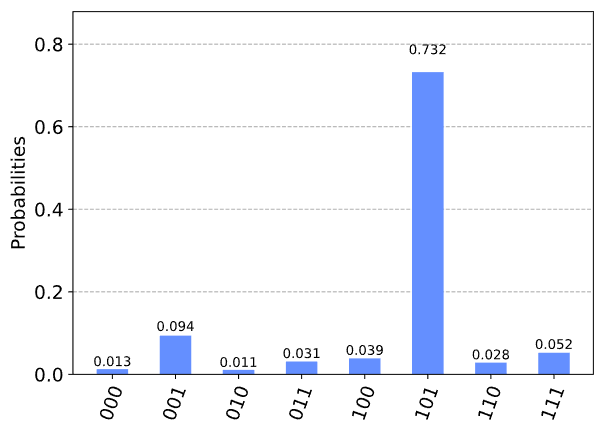## 9. 问题

1. 通过准备傅里叶状态 |\widetilde{5}\rangle 来测试上述QFT的实现，其中 \text{QFT}^{\dagger}|\widetilde{5}\rangle = |101\rangle 。尝试找到状态 |a\rangle ，使 \text{QFT}^{\dagger}|a\rangle = |100\rangle

2. 查找状态 |b\rangle ，使 \text{QFT}^{\dagger}|b\rangle = |011\rangle

3. 尝试编写没有递归的QFT函数。使用Qiskit的单一模拟器来验证您的结果。

## 10. 参考文献

1. M. Nielsen和I. Chuang，量子计算和量子信息，剑桥信息和自然科学系列(剑桥大学出版社，剑桥，2000)。

import qiskit.tools.jupyter

%qiskit_version_table